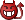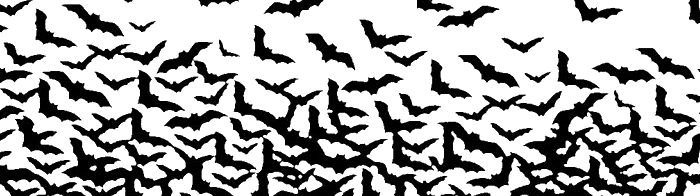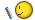1 knightbulletin Moderátor ekkor: 13 Jul 2018 [13:44] frissítve: 24 Jul 2018 [22:14] Megosztás: Translate»Dear our fearless Knights and Ladies! We invite you to take part in the contest 13-13-13, dedicated to the Friday the 13th.And now the conditions of competition: - Logs are accepted only from temporary locations. - In the report you can specify no more than 13 logs with compulsory research. - Temporary locations located inside the contour are not accepted! - Location "secret treasure" will not be considered. - All battle logs have to be in the above mentioned contest period of time. - In order to preserve you logs copy them to your computer. - Attentively check your report before sending! - Any fraud with logs will lead to disqualification - Sart of the contest: 13.07.2018 , 13-13 hours (server time). Finish 16.07.2018 , 13-13 hours (server time). - Each player can send only one report, reception will be ended 16.07.2018 , 23-00 hours (server time). Attention combat logs are accepted only in the form of report! To draw up your report, click on the picture:Nomination 1: “Davil's dozen” Prize: The player explore 13 (no more, no less) different types of locations. Prize: 1999 gold, 1666 diamonds, set of artefacts, resourses, unical units. Nomination 2: “AD/Hell's dozen”. Prize: The player explore 13 (no more, no less) different types of locations. Receive no less 66 diferent kinds of artefacts. Prize: 2333 gold, 1999 diamonds, hero + heroes skills + set of artefacts, resourses, unical units. Nomination 3: “13 infernal circles of hell”. Super Prize: The player explore 13 (no more, no less) different types of locations. Receive 13 diferent kinds of artefacts 13 pieces of each (not less!). Prize: 3666 gold, 2666 diamonds, hero + heroes skills + set of artefacts, resourses, unical units. Forward brave knights and beautiful ladies and not a step back! Good luck and have fun on exploring locations!1 PERCHA1966 ekkor: 16 Jul 2018 [02:34] percha1966 el dorado https://eldorado.1100ad.com/logger/ROB/1c990c82511e7ddd2c5ca5bac9e60a71/wicket:pageMapName/wicket-4 https://eldorado.1100ad.com/logger/ROB/15e1502e715323dcc6cab2b60c7781bc/wicket:pageMapName/wicket-6 https://eldorado.1100ad.com/logger/ROB/defdd809486cafad5c475bf17680dd21/wicket:pageMapName/wicket-8 https://eldorado.1100ad.com/logger/ROB/e42586b1da74c8b74f109041989fa8af/wicket:pageMapName/wicket-10 12https://eldorado.1100ad.com/logger/LOG/3ddf1735bea36af310a7fdcf91258447/wicket:pageMapName/wicket-15 https://eldorado.1100ad.com/logger/LOG/c35fc4061b2db94866a66ef9c8ad0210/wicket:pageMapName/wicket-5 https://eldorado.1100ad.com/logger/LOG/0eb5d9400e19e202f3435d49d516587b/wicket:pageMapName/wicket-16 https://eldorado.1100ad.com/logger/LOG/f85372f97fac3998521162151be2fa9e/wicket:pageMapName/wicket-17 https://eldorado.1100ad.com/logger/LOG/f1914dc275d34b872aef42169de15550/wicket:pageMapName/wicket-19 https://eldorado.1100ad.com/logger/LOG/5dd9e426c4b6f8f9b11b8242fb65b7a9/wicket:pageMapName/wicket-23 https://eldorado.1100ad.com/logger/ROB/4c9a7225cc98190943e30995f0dae1cf/wicket:pageMapName/wicket-24 https://eldorado.1100ad.com/logger/ROB/c25eb56d99284bbf72458c49f9809bf8/wicket:pageMapName/wicket-25 https://eldorado.1100ad.com/logger/ROB/3e790ee0a8b5c43fb94b2f8fe00e4735/wicket:pageMapName/wicket-27 knightbulletin Moderátor ekkor: 16 Jul 2018 [12:26] Attention combat logs are accepted only in the form of report! To draw up your report, click on the picture:- Competition extended! Finish 17.07.2018 , 13-13 hours (server time). - Each player can send only one report, reception will be ended 17.07.2018 , 23-00 hours (server time). knightbulletin Moderátor ekkor: 24 Jul 2018 [22:15] frissítve: 26 Jul 2018 [13:07] Congratulations to the winners of the contest: Friday the 13th!Reporting tableUnification of AragonNomination 1: bambalite (Number of logs: = 13 , Number of research: = 13, Quantity of the found artifacts: The number of different types of artifacts: = 23 , Number of artifacts (13) = 3) New BetaNomination 2: 1986HOHOL (Number of logs: = 13 , Number of research: = 13, Quantity of the found artifacts: The number of different types of artifacts: = 72 , Number of artifacts (13) = 2) Nomination 2: dnzory (Number of logs: = 13 , Number of research: = 13, Quantity of the found artifacts: The number of different types of artifacts: = 80 , Number of artifacts (13) = 4) Nomination 2: Daigucis1 (Number of logs: = 13 , Number of research: = 13, Quantity of the found artifacts: The number of different types of artifacts: = 78 , Number of artifacts (13) = 3) Nomination 3: SIZIF6 (Number of logs: = 13 , Number of research: = 13, Quantity of the found artifacts: The number of different types of artifacts: = 87 , Number of artifacts (13) = 17) Kingdom of BohemiaNomination 2: arikaro (Number of logs: = 13 , Number of research: = 13, Quantity of the found artifacts: The number of different types of artifacts: = 89 , Number of artifacts (13) = 11) Incentive prize: GLBUH (Number of logs: = 13 , Number of research: = 11, Quantity of the found artifacts: The number of different types of artifacts: = 80 , Number of artifacts (13) = 15) El DoradoNomination 2: Adler01 (Number of logs: = 13 , Number of research: = 13, Quantity of the found artifacts: The number of different types of artifacts: = 84 , Number of artifacts (13) = 4) Nomination 2: AlexKronin (Number of logs: = 13 , Number of research: = 13, Quantity of the found artifacts: The number of different types of artifacts: = 84 , Number of artifacts (13) = 9) Nomination 2: hunta (Number of logs: = 13 , Number of research: = 13, Quantity of the found artifacts: The number of different types of artifacts: = 80 , Number of artifacts (13) = 4) Nomination 3: percha1966 (Number of logs: = 13 , Number of research: = 13, Quantity of the found artifacts: The number of different types of artifacts: = 53 , Number of artifacts (13) = 30) EurasiaNomination 2: zils (Number of logs: = 13 , Number of research: = 13, Quantity of the found artifacts: The number of different types of artifacts: = 85 , Number of artifacts (13) = 8) Nomination 2: Daigucis123 (Number of logs: = 13 , Number of research: = 13, Quantity of the found artifacts: The number of different types of artifacts: = 78 , Number of artifacts (13) = 3) Nomination 2: burbon21 (Number of logs: = 13 , Number of research: = 13, Quantity of the found artifacts: The number of different types of artifacts: = 82 , Number of artifacts (13) = 6) Nomination 2: VIZANTIA (Number of logs: = 13 , Number of research: = 13, Quantity of the found artifacts: The number of different types of artifacts: = 79 , Number of artifacts (13) = 7) Nomination 2: Nidhoggr (Number of logs: = 13 , Number of research: = 13, Quantity of the found artifacts: The number of different types of artifacts: = 93 , Number of artifacts (13) = 6) Nomination 2: EgilsVanags (Number of logs: = 13 , Number of research: = 13, Quantity of the found artifacts: The number of different types of artifacts: = 79 , Number of artifacts (13) = 4) Gold RushNomination 2: Micerinos (Number of logs: = 13 , Number of research: = 13, Quantity of the found artifacts: The number of different types of artifacts: = 85 , Number of artifacts (13) = 5) Nomination 2: martins256 (Number of logs: = 13 , Number of research: = 13, Quantity of the found artifacts: The number of different types of artifacts: = 89 , Number of artifacts (13) = 9) Nomination 2: jraimis (Number of logs: = 13 , Number of research: = 13, Quantity of the found artifacts: The number of different types of artifacts: = 87 , Number of artifacts (13) = 7) Nomination 2: tato7 (Number of logs: = 13 , Number of research: = 13, Quantity of the found artifacts: The number of different types of artifacts: = 82 , Number of artifacts (13) = 3) Holy Grail SearchNomination 2: Margo Clarissima (Number of logs: = 13 , Number of research: = 13, Quantity of the found artifacts: The number of different types of artifacts: = 77 , Number of artifacts (13) = 4) Nomination 2: ternipe (Number of logs: = 13 , Number of research: = 13, Quantity of the found artifacts: The number of different types of artifacts: = 87 , Number of artifacts (13) = 6) IslagrandeNomination 2: Ludmila1 (Number of logs: = 13 , Number of research: = 13, Quantity of the found artifacts: The number of different types of artifacts: = 86 , Number of artifacts (13) = 8) Nomination 2: andreysssr2012 (Number of logs: = 13 , Number of research: = 13, Quantity of the found artifacts: The number of different types of artifacts: = 87 , Number of artifacts (13) = 10) Nomination 2: falikon (Number of logs: = 13 , Number of research: = 13, Quantity of the found artifacts: The number of different types of artifacts: = 88 , Number of artifacts (13) = 7) Nomination 2: Kubba (Number of logs: = 13 , Number of research: = 13, Quantity of the found artifacts: The number of different types of artifacts: = 91 , Number of artifacts (13) = 11) Nomination 2: wetryak (Number of logs: = 13 , Number of research: = 13, Quantity of the found artifacts: The number of different types of artifacts: = 79 , Number of artifacts (13) = 4) Nomination 2: alleksan77 (Number of logs: = 13 , Number of research: = 13, Quantity of the found artifacts: The number of different types of artifacts: = 72 , Number of artifacts (13) = 2) Nomination 2: bazi666 (Number of logs: = 13 , Number of research: = 13, Quantity of the found artifacts: The number of different types of artifacts: = 82 , Number of artifacts (13) = 6) Nomination 3: Ready_To_Fight (Number of logs: = 13 , Number of research: = 13, Quantity of the found artifacts: The number of different types of artifacts: = 87 , Number of artifacts (13) = 15) Koryo - Rise of EmpireNomination 2: uawest (Number of logs: = 13 , Number of research: = 13, Quantity of the found artifacts: The number of different types of artifacts: = 84 , Number of artifacts (13) = 8) Nomination 2: Fox118 (Number of logs: = 13 , Number of research: = 13, Quantity of the found artifacts: The number of different types of artifacts: = 88 , Number of artifacts (13) = 9) Nomination 2: ARBURUA (Number of logs: = 13 , Number of research: = 13, Quantity of the found artifacts: The number of different types of artifacts: = 90 , Number of artifacts (13) = 10) Nomination 2: 27adebayor27 (Number of logs: = 13 , Number of research: = 13, Quantity of the found artifacts: The number of different types of artifacts: = 89 , Number of artifacts (13) = 9) Nomination 2: Lapsite (Number of logs: = 13 , Number of research: = 13, Quantity of the found artifacts: The number of different types of artifacts: = 85 , Number of artifacts (13) = 10) Nomination 2: Javdet (Number of logs: = 13 , Number of research: = 13, Quantity of the found artifacts: The number of different types of artifacts: = 81 , Number of artifacts (13) = 5) Livonian OrderNomination 1: martkita (Number of logs: = 13 , Number of research: = 13, Quantity of the found artifacts: The number of different types of artifacts: = 57 , Number of artifacts (13) = 4) Nomination 2: dainis070 (Number of logs: = 13 , Number of research: = 13, Quantity of the found artifacts: The number of different types of artifacts: = 84 , Number of artifacts (13) = 9) Nomination 2: fyvm4y (Number of logs: = 13 , Number of research: = 13, Quantity of the found artifacts: The number of different types of artifacts: = 93 , Number of artifacts (13) = 12) Nomination 2: Tainbow (Number of logs: = 13 , Number of research: = 13, Quantity of the found artifacts: The number of different types of artifacts: = 84 , Number of artifacts (13) = 7) Nomination 3: stepnina (Number of logs: = 13 , Number of research: = 13, Quantity of the found artifacts: The number of different types of artifacts: = 87 , Number of artifacts (13) = 15) Incentive prize: tato7 (Number of logs: = 13 , Number of research: = 12, Quantity of the found artifacts: The number of different types of artifacts: = 83 , Number of artifacts (13) = 8) MidgardNomination 2: DURR (Number of logs: = 13 , Number of research: = 13, Quantity of the found artifacts: The number of different types of artifacts: = 81 , Number of artifacts (13) = 4) Nomination 2: alex1662 (Number of logs: = 13 , Number of research: = 13, Quantity of the found artifacts: The number of different types of artifacts: = 85 , Number of artifacts (13) = 3) Nomination 2: kalex61 (Number of logs: = 13 , Number of research: = 13, Quantity of the found artifacts: The number of different types of artifacts: = 91 , Number of artifacts (13) = 5) Nomination 2: liuciperis (Number of logs: = 13 , Number of research: = 13, Quantity of the found artifacts: The number of different types of artifacts: = 76 , Number of artifacts (13) = 6) Nomination 3: GOTHOB (Number of logs: = 13 , Number of research: = 13, Quantity of the found artifacts: The number of different types of artifacts: = 86 , Number of artifacts (13) = 16) NanbanNomination 2: mmm54 (Number of logs: = 13 , Number of research: = 13, Quantity of the found artifacts: The number of different types of artifacts: = 90 , Number of artifacts (13) = 10) Incentive prize: Avmenida (Number of logs: = 12 , Number of research: = 12, Quantity of the found artifacts: The number of different types of artifacts: = 85 , Number of artifacts (13) = 6) atlantys (Number of logs: = 13 , Number of research: = 12, Quantity of the found artifacts: The number of different types of artifacts: = 83 , Number of artifacts (13) = 3) [color=#0E003C] Outremer [b] Nomination 2: daigucis1 (Number of logs: = 13 , Number of research: = 13, Quantity of the found artifacts: The number of different types of artifacts: = 77 , Number of artifacts (13) = 5) Nomination 2: danunach (Number of logs: = 13 , Number of research: = 13, Quantity of the found artifacts: The number of different types of artifacts: = 84 , Number of artifacts (13) = 5) Nomination 2: MACGREGORS (Number of logs: = 13 , Number of research: = 13, Quantity of the found artifacts: The number of different types of artifacts: = 88 , Number of artifacts (13) = 12) Nomination 2: GRABLY (Number of logs: = 13 , Number of research: = 13, Quantity of the found artifacts: The number of different types of artifacts: = 90 , Number of artifacts (13) = 11) Nomination 2: PERRI (Number of logs: = 13 , Number of research: = 13, Quantity of the found artifacts: The number of different types of artifacts: = 89 , Number of artifacts (13) = 11) Nomination 2: recycler1988 (Number of logs: = 13 , Number of research: = 13, Quantity of the found artifacts: The number of different types of artifacts: = 87 , Number of artifacts (13) = 6) Nomination 2: pterm (Number of logs: = 13 , Number of research: = 13, Quantity of the found artifacts: The number of different types of artifacts: = 75 , Number of artifacts (13) = 3) Nomination 3: arnoldsw22 (Number of logs: = 13 , Number of research: = 13, Quantity of the found artifacts: The number of different types of artifacts: = 91 , Number of artifacts (13) = 16) Incentive prize: alex1662 (Number of logs: = 12 , Number of research: = 12, Quantity of the found artifacts: The number of different types of artifacts: = 88 , Number of artifacts (13) = 15) Ready_To_Fight ekkor: 26 Jul 2018 [08:04] frissítve: 30 Jul 2018 [12:42] I had 13 artifacts 13 pieces each, it took a long time to get this, you never said it had to be 13 of one art in one camp....Those who did as I did, deserve prize 3, not 2...You were not clear Nomination 3: Ready_To_Fight (Number of logs: = 13 , Number of research: = 13, Quantity of the found artifacts: The number of different types of artifacts: = 87 , Number of artifacts (13) = 15) 1